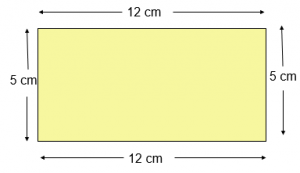# Perimeter of Rectangle

Perimeter of Rectangle is equal to twice of Sum of its length and breadth.

Perimeter of Rectangle Formula = 2 ( Length of Rectangle + Breadth of Rectangle )

## Perimeter of Rectangle Examples

Find the perimeter of a rectangle in which:

Example 1

length = 12 cm and  breadth = 5 cm

Explanation:Given:
Length = 12 cm
Perimeter of Rectangle = 2 ( l + b ) units
= 2 ( 12 + 5 ) cm
= ( 2 x 17 ) cm
= 34 cm

Example 2

length = 7 cm and breadth = 11 cm

Explanation:

Given:
Length = 7 cm
Perimeter of rectangle = 2 ( l + b ) units
= 2 ( 7 + 11 ) cm (Here, Length = 7 cm , Breadth = 11 cm )
= ( 2 x 18 ) cm
= 36 cm

Example 3

length = 25 cm and breadth = 12 cm

Explanation:

Given:
Length = 25 cm
Perimeter of rectangle = 2 ( l + b ) units
= 2 ( 25 + 12 ) cm (Here, Length = 25 cm , Breadth = 12 cm )
= ( 2 x 37 ) cm
= 74 cm

Example 4

length = 21 cm and breadth = 7 cm

Explanation:

Given:
Length = 21 cm
Perimeter of rectangle = 2 ( l + b ) units
= 2 ( 21 + 7 ) cm (Here, Length = 21 cm , Breadth = 7 cm )
= ( 2 x 28 ) cm
= 56 cm

Example 5

length = 16 cm and breadth = 9 cm

Explanation:

Given:
Length = 16 cm